## Analytical Chemistry

Learn the toughest concepts covered in your Analytical Chemistry class with step-by-step video tutorials and practice problems.

4 & 5. Statistics, Quality Assurance and Calibration Methods

# The Gaussian Distribution

Performing an experiment numerous times with no systematic error results in a smooth curve called the Gaussian Distribution.

The Gaussian Distribution & Z-Table
1
concept

## Gaussian Distribution4m
Play a video: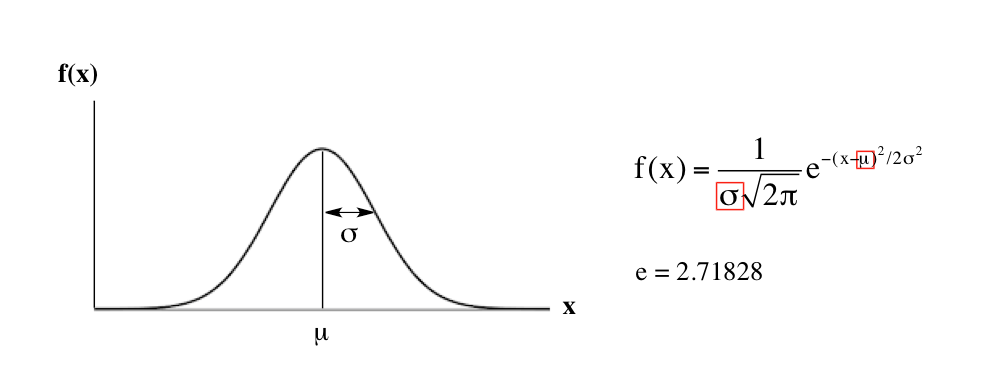2
example

## Gaussian Distribution7m
Play a video: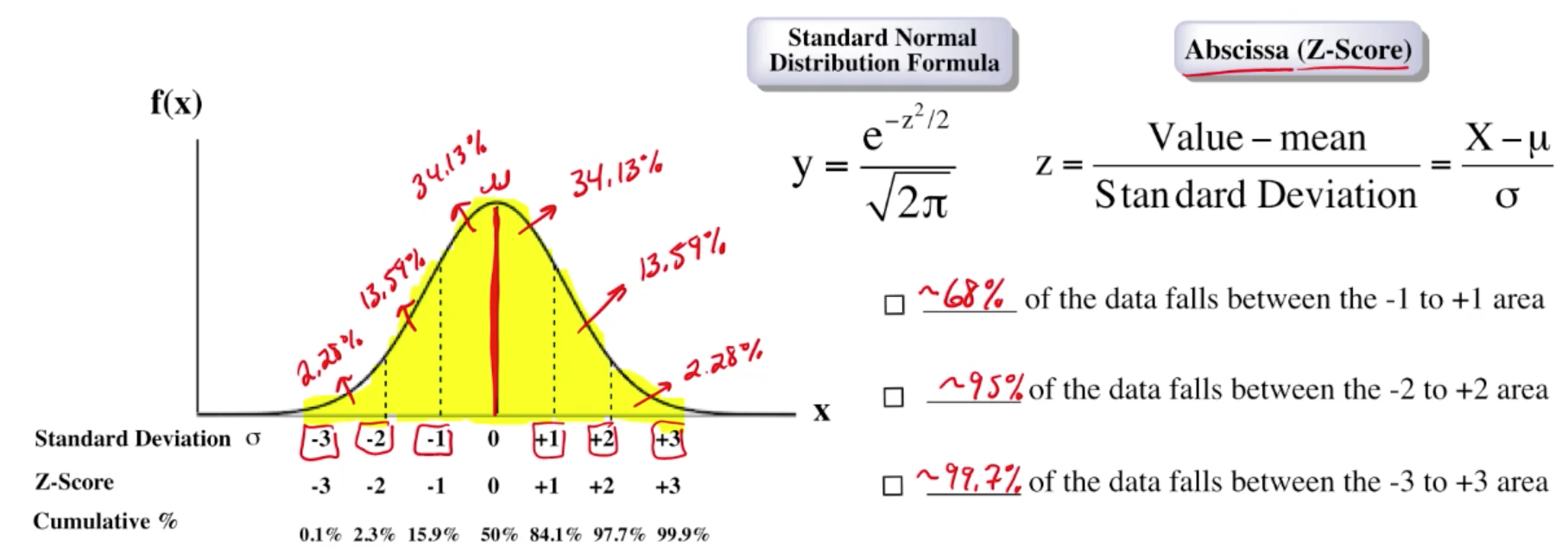3
example

## The Gaussian Distribution & Z-Table2m
Play a video:
So the Gaussian distribution curve gives us the probability of a percentage of a population falling within certain parameters. When we're given a Z score now to find the scores we use Z tables Now remember on the previous page that Z equals the value that we're looking at minus are mean or population mean divided by our standard deviation. Now in this image we have a Z score here Here, let's say that that Z score equals negative 1.65. Remember all the numbers to the left of zero will be negative. All the numbers to the right will be positive. Using the Z score table here we have negative 33.4 which is around here all the way to zero. So this Z table looks at the negative values for Z. Here we have a score of negative 1.65, realize here that when it comes to the Z scores here we're looking at the first two digits and then here these numbers helped to give us our third digit. Okay, So we have negative 1.65. So negative 1.6 is here And then we just have to pop find .05 which is right here and then we see where they meet. So both of them meet right here. So that's gonna give us our p value which is our probability for the percentage of the population that's gonna fit within those parameters. So here p equals .0495. So what does that mean? That's the decimal form? So we'd multiply it by 100. So that would be 4.95%. So what that's telling us here is that if we were to shade in this portion here, It means that 4.95% would fall within those parameters. From negative 3.4 up to negative 1.6 here. It's not drawn to scale Because it looks like that percentage is bigger than 4.95%. But again, I just chose an arbitrary number. I just chose negative 1.65. Just to show how we'd use the Z table in order to figure out what our probability would be. Remember. This first portion looks at the negative aspect of the Gaussian distribution curve, click on the next video and see what we would do when we're looking at Z scores that are to the right of my population mean of zero. We employ the same method, but let's just see what would happen in those cases.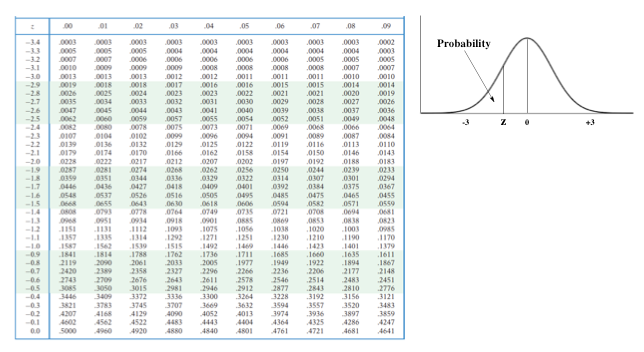4
example

## The Gaussian Distribution & Z-Table2m
Play a video:
So here we have a Z score that now falls To the right of my population mean of zero. Now let's just come up with an arbitrary number again. So we'll say Z here equals 1.82 for that value. So remember the first two digits from my Z score are found here in this column. So we have to look for 1.8 And then the third digit, the two we look here at .02, we'd see where they would meet up. So they'd meet up here. That means that my probability p equals .9656. So that would mean times 196.56%. So that means here that if we're taking a consideration all the negative portion of my Gaussian distribution curve plus this portion up to Z. That would represent 96.56% of my entire population. Now besides that, what if they were to ask me um what is the probability if we're looking at Z equals zero to Z equals 1.82. In that case we'd only be looking at this portion of my Gaussian distribution curve. Okay, so we'd only be looking at this portion here. Now remember the mean represents the exact center of my Gaussian distribution curve? That would mean that this portion here represents 50 And then the other half represents 50%. So we're not looking at the 50%, the part that's the left of my population mean. So all we have to do to figure out what's the percentage between these two Z values is just do 96.56% -50%. And that would give me 46.56% of the population would fall between a Z score of 0 to Z score of 1.82. So again, just remember, in order to determine the probability of a population falling within certain parameters, we have to find our Z. Score and then compare it to our Z. Table in order to find our value P, which represents the probability the percentage that we're looking for.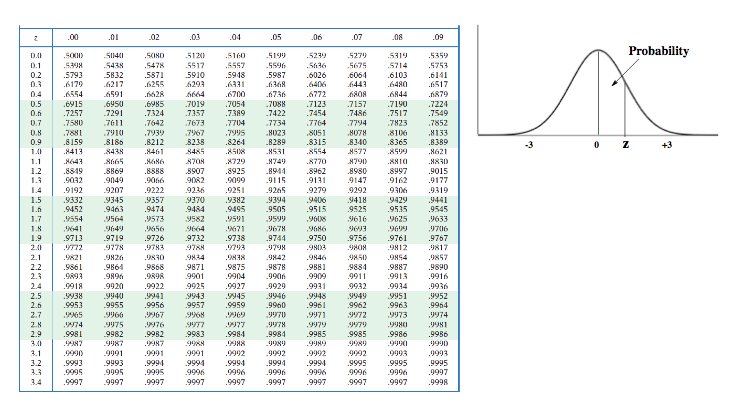The Gaussian Distribution & Z-Tables Calculations
5
example

## Gaussian Distribution & Z-Table3m
Play a video: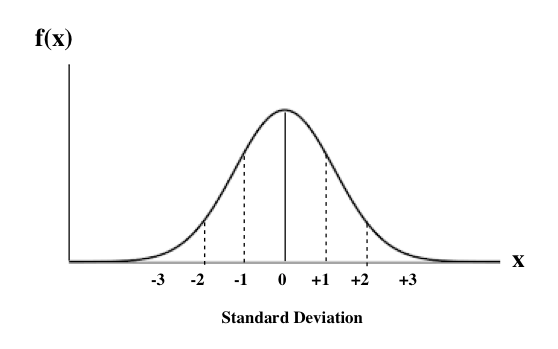6
example

## Gaussian Distribution & Z-Table2m
Play a video: